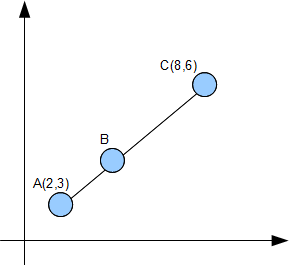## Posts

Showing posts from August, 2010

### Completing the SquareCompleting the square is a technique required to find equations of circle Example 1

### Coordinate Geometry Part IIExample 1 A typical coordinate geometry question from the O Levels Given that AC = 3AB, find the coordinates of point B. Remarks: A lot of students will try to use the distance formula to form simultaneous equations in x and y to find the coordinates of B. This is a very long and tedious method. A much simpler method is to use ratio and proportion to find the coordinates of B To find the x coordinate of B We can use the same method to find the y coordinate of point B Therefore the coordinates of point B is (4,4)

### Coordinate Geometry Part ITechnique 1: Finding the gradient of a line Parallel lines Parallel lines have the same gradient Perpendicular lines If a line has a gradient of 1/2 , the gradient of the line perpendicular to it is -2. -2 is the negative reciprocal of 1/2. In other words, we "flip" the fraction 1/2 to get 2 and attach a negative sign Technique 2: Finding equation of a straight line The general format of a straight line is y = mx + c where m is the gradient and, c is the y intercept Example 2 Find the equation of a line that contains the points (1,2) and (3,4). From the example above we know that gradient of the line is 1. Hence the equation of the line is: y = 1x + c To find the y intercept, c, we sub in one of the points I choose the point (1,2) and sub x =1, y = 2 2 = 1 + c Hence c = 1 The equation of the line is y = x + 1 Let us combine the techniques we have learnt so far to solve a typical O Level question Question 1 The figure above shows a trapezium where AB is parallel to CD. Both

### Roots of Quadratic Equation Part II